• MATLAB 给函数加上随机噪声/扰动 在进行数值计算时，为了检验一个数值算法是否稳定，通常要给测量数据加上随机噪声。假设需要添加噪声的函数是f(x,t)=(1+t)2sin⁡2(2πx)f(x,t)=(1+t)^2\sin^2(2\pi x)f(x,t)=(1+t)2...
在进行数值计算时，为了检验一个数值算法是否稳定，通常要给测量数据加上随机噪声。假设需要添加噪声的函数是

f

(

x

,

t

)

=

(

1

+

t

)

2

sin

⁡

2

(

2

π

x

)

f(x,t)=(1+t)^2\sin^2(2\pi x)

，那么添加噪声的方法是：

f

δ

(

x

i

,

t

j

)

=

f

(

x

i

,

t

j

)

+

δ

(

2

R

i

j

−

1

)

f

(

x

i

,

t

j

)

.

f^\delta(x_i,t_j)=f(x_i,t_j)+\delta (2R_{ij}-1)f(x_i,t_j).

其中

f

δ

(

x

i

,

t

j

)

f^\delta(x_i,t_j)

是添加了噪声的数据，

δ

\delta

是相对误差水平，

R

i

j

R_{ij}

是在区间

[

0

,

1

]

[0,1]

上均匀分布的随机数。 下面我们想给

f

(

x

,

t

)

f(x,t)

加上相对误差水平为0.01的噪声，MATLAB中可利用如下代码实现：
% % 定义函数f(x,t)
f=@(x,t) (1+t)^2*sin(2*pi*x).^2);
% % 添加噪声
f_delta=@(x,t) f(x,t)+0.01*(2*rand(size(F))-1)*f(x,t); %size(F)为生成的带噪声的向量或矩阵的大小

展开全文• 给一组数据添加低幅值不同频率的正弦函数的扰动，从而产生多组数据
• 有时我们需要对某种顺序作出随机打乱的效果，这时在MATLAB中的一个函数randperm就显得非常有用处。它的用法很简单，举个例子：如果想产生一个1到24的随机顺序，那么命令randperm(24)会告诉你一个：[24 8 21 15 2...
有时我们需要对某种顺序作出随机打乱的效果，这时在MATLAB中的一个函数
randperm就显得非常有用处。它的用法很简单，举个例子：如果想产生一个1到24的随机顺序，那么命令randperm(24)会告诉你一个：
[24 8 21 15 2 22 16 19 10 7 4 3 11 14 6 12 23 9 5 20 18 13 17 1]

展开全文• 基于拟牛顿法原理,结合同时扰动随机逼近算法特性提出了一种搜索方向dk的计算方法,从而提高了同时扰动随机逼近算法的收敛速度和逼近精度.针对典型优化问题分别比较了改进后的同时扰动随机逼近算法、标准同时扰动随机...
• 【优化求解】基于自然选择和随机扰动改进磷虾群算法matlab源码.md
• 使用matlab计算随机变量的期望和方差，以及随机变量函数的期望和方差
• WeaGETS是一个基于Matlab的多功能随机每日天气生成器（WeaGETS），用于产生无限长的每日降水量，最高和最低温度（Tmax和Tmin）系列，从而可以对罕见的气象变量事件进行影响研究。 此外，它可以通过扰动参数来解释...
• 研究了具有混合时滞的混沌模糊细胞神经网络的同步问题。 通过脉冲积分微分不等式和伊藤公式，获得了一些足以使网络在脉冲和随机扰动下同步的充分判据。 给出了算例和仿真，以证明所提出结果的效率和优势。研究论文
• 1.MATLAB赋值语句 （1）变量=表达式 （2）表达式 其中表达式是用运算符将有关运算量连接起来的式子，其结果是一个矩阵 例如计算表达式的值，并显示计算结果，在MATLAB命令窗口输入指令： 其中pi和i都是MATLAB...
1.MATLAB赋值语句
（1）变量=表达式
（2）表达式
其中表达式是用运算符将有关运算量连接起来的式子，其结果是一个矩阵
例如计算表达式的值，并显示计算结果，在MATLAB命令窗口输入指令：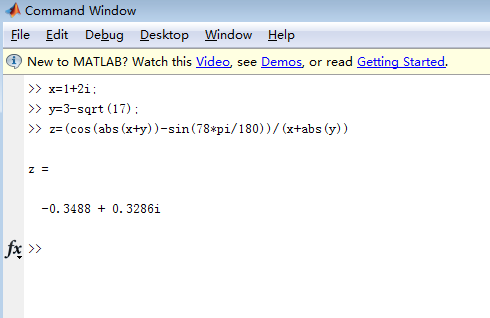其中pi和i都是MATLAB预先定义的变量分别代表圆周率π和虚数单位。
2.MATLAB矩阵
（2.1）矩阵的建立
直接输入法：最简单的建立矩阵的方法是从键盘直接输入矩阵的元素。具体方法如下：将矩阵的元素用方括号括起来，按矩阵行的顺序输入各元素，同一行的各元素之间用空格或逗号分隔，不同行的元素之间用分号分隔。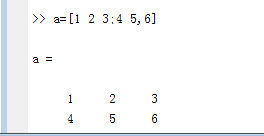利用M文件建立矩阵：对于比较大且比较复杂的矩阵，可以为它专门建立一个M文件。下面通过一个简单例子来说明如何利用M文件创建矩阵。
i：启动有关编辑程序或MATLAB文本编辑器，并输入待建矩阵：file-new-scriptii ：把输入的内容以纯文本方式存盘(设文件名为mymatrix.m)，这里一定要有function语句。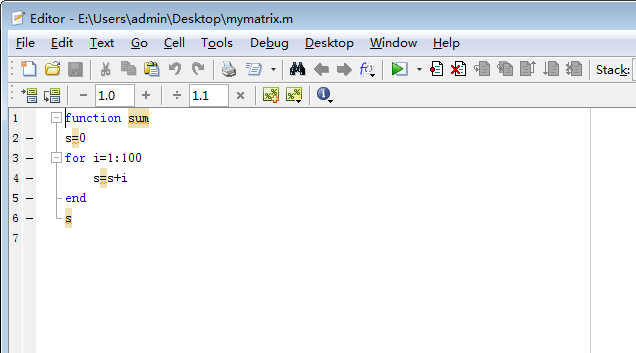iii： 在workspace中替换文件位置在MATLAB命令窗口中输入mymatrix，即运行该M文件，就会自动建立一个名为MYMAT的矩阵，可供以后使用。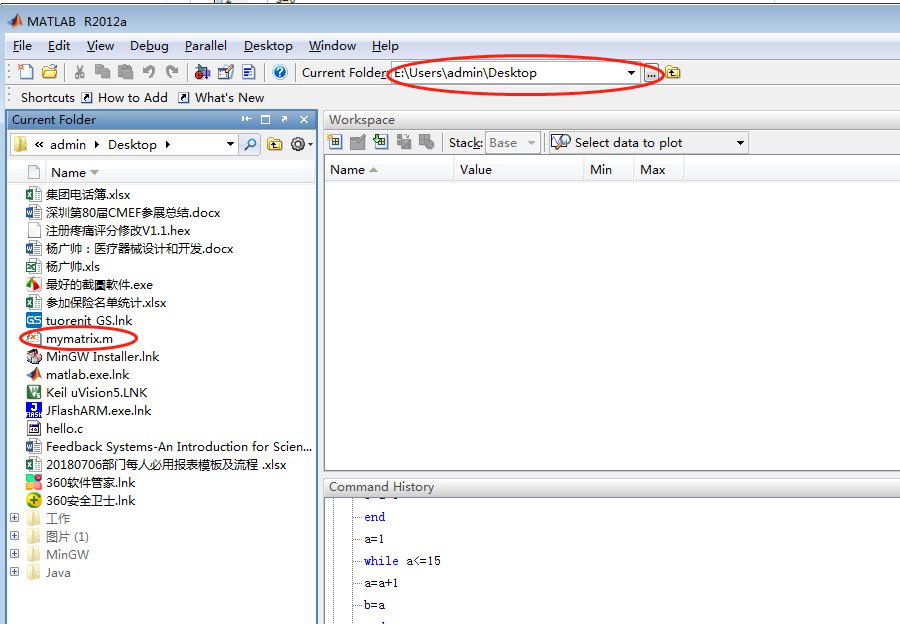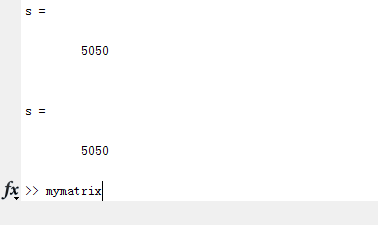3.特殊矩阵
常见的特殊矩阵函数有zeros：产生全0矩阵（零矩阵）；ones：产生全1矩阵（幺矩阵）；eye：产生单位矩阵；rand：产生0~1间均匀分布的随机矩阵；randn：产生均值为0，方差为1的标准正态分布随机矩阵。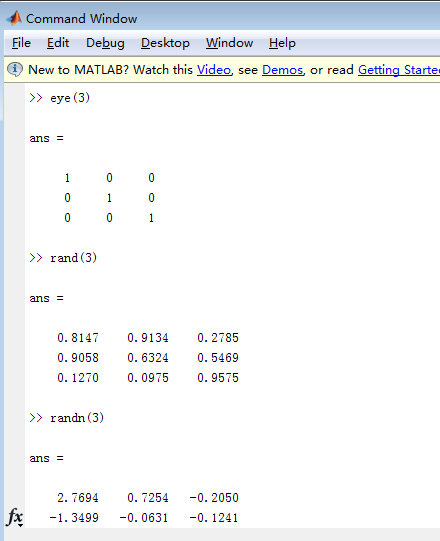魔方矩阵：有一个有趣的性质，其每行、每列及两条对角线上的元素和都相等。对于n阶魔方阵，其元素由1,2,3,…,n2共n2个整数组成。MATLAB提供了求魔方矩阵的函数magic(n)，其功能是生成一个n阶魔方阵。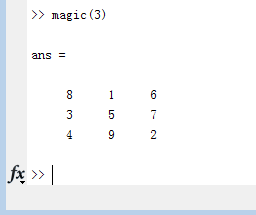范得蒙(Vandermonde)矩阵最后一列全为1，倒数第二列为一个指定的向量，其他各列是其后列与倒数第二列的点乘积。可以用一个指定向量生成一个范得蒙矩阵。在MATLAB中，函数vander(V)生成以向量V为基础向量的范得蒙矩阵。例如，A=vander([1;2;3;5])即可得到上述范得蒙矩阵。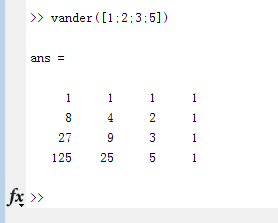在MATLAB中，生成希尔伯特矩阵的函数是hilb(n)。 使用一般方法求逆会因为原始数据的微小扰动而产生不可靠的计算结果。MATLAB中，有一个专门求希尔伯特矩阵的逆的函数invhilb(n)，其功能是求n阶的希尔伯特矩阵的逆矩阵。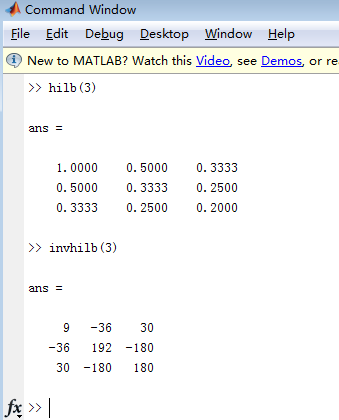托普利兹(Toeplitz)矩阵除第一行第一列外，其他每个元素都与左上角的元素相同。生成托普利兹矩阵的函数是toeplitz(x,y)，它生成一个以x为第一列，y为第一行的托普利兹矩阵。这里x, y均为向量，两者不必等长。toeplitz(x)用向量x生成一个对称的托普利兹矩阵。例如T=toeplitz(1:6)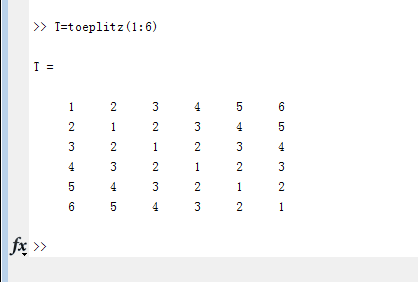MATLAB生成伴随矩阵的函数是compan(p)，其中p是一个多项式的系数向量，高次幂系数排在前，低次幂排在后。例如，为了求多项式的x3-7x+6的伴随矩阵，可使用命令：p=[1,0,-7,6]; compan(p)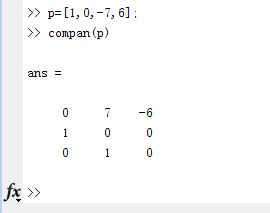我们知道，二次项(x+y)n展开后的系数随n的增大组成一个三角形表，称为杨辉三角形。由杨辉三角形表组成的矩阵称为帕斯卡(Pascal)矩阵。函数pascal(n)生成一个n阶帕斯卡矩阵。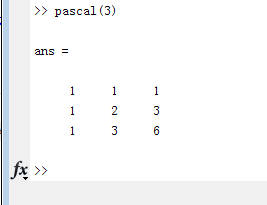展开全文• 这些文件包含基本的随机预测控制仿真器，用于带有加性扰动的多变量线性系统。 干扰具有高斯概率分布并且可以有界。 总共有两个模拟器：基于状态机会约束的MPC模拟器； 另一种基于实现干扰的场景。 另外，对于每个...
• ## 粒子群优化算法(PSO)-MATLAB代码

千次阅读 热门讨论 2019-07-16 11:35:26
粒子群优化算法(PSO)-MATLAB代码 关于粒子群优化算法(PSO)的介绍与一种C++实现可以参考链接： PSO介绍及其一种C++实现 ，这里不再赘述。 本片博文目的在于提供并简要介绍一种粒子群优化算法(PSO)的MATLAB代码实现...
粒子群优化算法(PSO)-MATLAB代码
关于粒子群优化算法(PSO)的介绍与一种C++实现可以参考链接： PSO介绍及其一种C++实现 ，这里不再赘述。
本片博文目的在于提供并简要介绍一种粒子群优化算法(PSO)的MATLAB代码实现。
本文提供的MATLAB代码中，PSO算法本身被封装成一个函数，优化目标函数的句柄作为PSO的输入参数，从而成为了一个较高独立性的函数模块。
以下为pso算法对应的函数输入输出说明。
%%%%%%%%%%%%%%%%%%%%%%%%%%%%%%%%%%%%%%%%%%%%%%%%%%
%函数名：zpso
%函数描述：粒子群优化算法函数
%输入参数：
%   objfunction：最大化目标函数句柄，目标函数是向量到实数的一个多元函数（定义域为超立方）
%   option：规定了pso算法的控制参数，利用ZPSO_parameters函数生成
%   evolutiontimes：粒子群进化代数限制
%输出参数：
%   bestPosition：搜索得到的目标函数最大值点
%   bestFitness：搜索得到的目标函数最大值
%%%%%%%%%%%%%%%%%%%%%%%%%%%%%%%%%%%%%%%%%%%%%%%%%%
function [bestPosition,bestFitness] = zpso(objfunction,option,evolutiontimes)

zpso函数的输入输入在注释中已经表述得较清楚，不再赘述，需要注意的是objfunction是一个以列向量为输入，标量为输出的目标函数。 以下介绍pso算法控制参数结构体——option 的生成。
%%%%%%%%%%%%%%%%%%%%%%%%%%%%%%%%%%%%%%%%%%%%%%%%%%
%函数名：zpso_parameters
%函数描述：生成PSO算法所需要的参数结构体
%输入参数：
%   dimension：候选解维度
%   minVal：候选解下界，列向量形式
%   maxVal：候选解上界，列向量形式
%   particalCount：粒子数量
%   maxSpeed：粒子最大运动速度
%   globalGuideCoe：全局最优引导加速度因子
%   localGuideCoe：个体最优引导加速度因子
%   disturbanceRate：粒子速度扰动概率
%   maxDisturbanceSpeed：最大扰动速度
%输出参数：
%   op：PSO算法所需参数结构体
%%%%%%%%%%%%%%%%%%%%%%%%%%%%%%%%%%%%%%%%%%%%%%%%%%
function op = zpso_parameters(dimension,minVal,maxVal,particalCount,maxSpeed,...
globalGuideCoe,localGuideCoe,...
disturbanceRate,maxDisturbanceSpeed)

需要说明的是 disturbanceRate 与 maxDisturbanceSpeed 参数。为了提高算法局部搜索能力，避免加速度因子的选择要求过于严苛，本文实现的pso算法中，粒子运动过程中有一定概率获得一个随机速度。 disturbanceRate 描述了粒子获得随机速度的概率，当其为0，算法退化为最基本的pso算法。而 maxDisturbanceSpeed 则限定了随机速度的最大值。
PSO算法的运行过程如下所示。其中红点代表当前粒子群搜索得到的全局最优解。黑色箭头代表了粒子位置及其运动速度。待优化函数为：

z

=

s

i

n

(

x

2

+

y

2

)

/

x

2

+

y

2

z = sin(\sqrt{x^2+y^2})/\sqrt{x^2+y^2}

运行效果：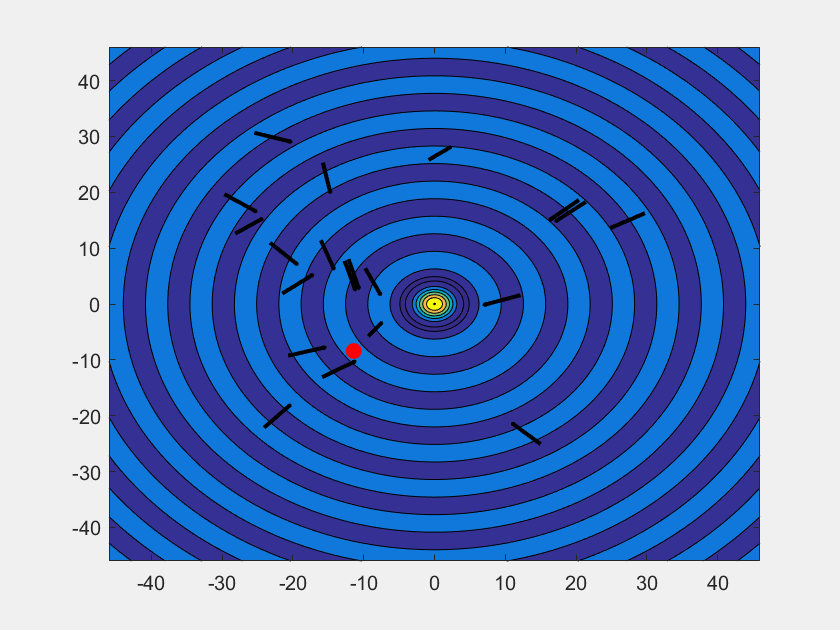以下附上与PSO算法相关的MATLAB代码。 <main.m> ——用于测试pso算法：
pso_op = zpso_parameters(2,[-10;-10],[10;10],200,4,1,1,0.1,2);
[bestPosition,bestFitness] = zpso(@objfunction,pso_op,100);

<objfunction.m>——用于定义优化目标函数
function y = objfunction(x)
x = x - [0.8;-0.4];
y = sin(sqrt(x'*x))/(sqrt(x'*x)+eps);
end

<zpso_parameters.m>——用于生成pso算法控制参数结构体
%%%%%%%%%%%%%%%%%%%%%%%%%%%%%%%%%%%%%%%%%%%%%%%%%%
%函数名：zpso_parameters
%函数描述：生成PSO算法所需要的参数结构体
%输入参数：
%   dimension：候选解维度
%   minVal：候选解下界，列向量形式
%   maxVal：候选解上界，列向量形式
%   particalCount：粒子数量
%   maxSpeed：粒子最大运动速度
%   globalGuideCoe：全局最优引导加速度因子
%   localGuideCoe：个体最优引导加速度因子
%   disturbanceRate：粒子速度扰动概率
%   maxDisturbanceSpeed：最大扰动速度
%输出参数：
%   op：PSO算法所需参数结构体
%%%%%%%%%%%%%%%%%%%%%%%%%%%%%%%%%%%%%%%%%%%%%%%%%%
function op = zpso_parameters(dimension,minVal,maxVal,particalCount,maxSpeed,...
globalGuideCoe,localGuideCoe,...
disturbanceRate,maxDisturbanceSpeed)
%粒子群维度
op.dimension = dimension;
%粒子位置下界
op.minVal = minVal;
%粒子位置上界
op.maxVal = maxVal;
%粒子个数
op.particalCount = particalCount;
%粒子最大速度
op.maxSpeed = maxSpeed;
%全局最优加速度因子
op.globalGuideCoe = globalGuideCoe;
%个体最优加速度因子
op.localGuideCoe = localGuideCoe;
%粒子速度扰动概率
op.disturbanceRate = disturbanceRate;
%粒子最大扰动速度
op.maxDisturbanceSpeed = maxDisturbanceSpeed;
end


<zpso.m>——用于实现pso算法
%%%%%%%%%%%%%%%%%%%%%%%%%%%%%%%%%%%%%%%%%%%%%%%%%%
%函数名：zpso
%函数描述：粒子群优化算法函数
%输入参数：
%   objfunction：最大化目标函数句柄，目标函数是向量到实数的一个多元函数（定义域为超立方）
%   option：pso算法参数，利用ZPSO_parameters函数生成
%   evolutiontimes：粒子群进化代数限制
%输出参数：
%   bestPosition：搜索得到的目标函数最大值点
%   bestFitness：搜索得到的目标函数最大值
%%%%%%%%%%%%%%%%%%%%%%%%%%%%%%%%%%%%%%%%%%%%%%%%%%
function [bestPosition,bestFitness] = zpso(objfunction,option,evolutiontimes)

%% 获取pso算法参数
dimension = option.dimension;
minVal = option.minVal;
maxVal = option.maxVal;
particalCount = option.particalCount;
globalGuideCoe = option.globalGuideCoe;
localGuideCoe = option.localGuideCoe;
maxSpeed = option.maxSpeed;
disturbanceRate = option.disturbanceRate;
maxDisturbanceSpeed = option.maxDisturbanceSpeed;

%% 初始化粒子群
%初始化粒子位置，在[minVal,maxVal]超立方空间内
pso_position = minVal*ones(1,particalCount);
pso_position = pso_position+rand(dimension,particalCount).*((maxVal-minVal)*ones(1,particalCount));
%初始化粒子速度
pso_velocity = rand(dimension,particalCount); %生成随机速度
temp = ones(dimension,1) * sqrt(diag(pso_velocity' * pso_velocity))'; %求取随机速度模长
pso_velocity = pso_velocity./temp; %随机速度模长归一化
velocityMod = maxSpeed*ones(dimension,1)*rand(1,particalCount); %初始化速度大小随机
pso_velocity = velocityMod.*pso_velocity;
%初始化粒子适应度、最佳适应度与个体最优解位置
pso_fitness = zeros(1,particalCount);
for j = 1:particalCount
pso_fitness(j) = objfunction(pso_position(:,j));
end
pso_bestposition = pso_position;
pso_bestfitness = pso_fitness;
%更新全局最优适应度与最优解位置
[pso_globalbestfitness,temp] = max(pso_bestfitness);
pso_globalbestposition = pso_position(:,temp);
%% 粒子群进化
for generationCount = 1:evolutiontimes
%全局最优引导
pso_velocity = pso_velocity+globalGuideCoe*ones(dimension,1)*rand(1,particalCount)...
.*(pso_globalbestposition*ones(1,particalCount) - pso_position);
%局部最优引导
pso_velocity = pso_velocity+localGuideCoe*ones(dimension,1)*rand(1,particalCount)...
.*(pso_bestposition - pso_position);
%速度扰动
disturbanceVelocity = rand(dimension,particalCount);
temp = ones(dimension,1)*sqrt(diag(disturbanceVelocity'*disturbanceVelocity))';
disturbanceVelocity = disturbanceVelocity./temp;
temp = maxDisturbanceSpeed*ones(dimension,1)*rand(1,particalCount);
temp = temp .* (ones(dimension,1)*(rand(1,particalCount)<disturbanceRate));
disturbanceVelocity = temp.*disturbanceVelocity;
pso_velocity = pso_velocity+disturbanceVelocity;
%速度受限
temp = ones(dimension,1)*sqrt(diag(pso_velocity'*pso_velocity))';
pso_velocity = pso_velocity./temp;
temp = min(temp,maxSpeed);
pso_velocity = temp.*pso_velocity;
%位置更新
pso_position = pso_position + pso_velocity;
%位置受限
pso_position = max(pso_position,minVal*ones(1,particalCount));
pso_position = min(pso_position,maxVal*ones(1,particalCount));
%适应度更新
for j = 1:particalCount
pso_fitness(j) = objfunction(pso_position(:,j));
end
%最佳适度更新
index = pso_fitness>pso_bestfitness;
pso_bestfitness = max(pso_fitness,pso_bestfitness);
pso_bestposition(:,index) = pso_position(:,index);
%全局最优更新
[bestfitness,index] = max(pso_bestfitness);
index = index(1);
if(bestfitness > pso_globalbestfitness)
pso_globalbestfitness = bestfitness;
pso_globalbestposition = pso_bestposition(:,index);
end
end
%% 输出搜索结果
bestPosition = pso_globalbestposition;
bestFitness = pso_globalbestfitness;
end


受作者水平所限，matlab代码可能不尽如人意，仅用于记录、学习与分享。
展开全文粒子群优化算法
• 初勘数据处理之预测算法（随机森林random forest） 集成学习方法 集成学习方法，是整合多个分类方法的预测结果，以提高分类准确率。集成学习方法先用训练数据构建一组基分类器，再对所有基分类器的预测进行投票，...机器学习
• 文章目录一、理论基础1、磷虾群算法(KH)2、改进的磷虾群算法(ANRKH)（1）觅食权重和运动权重的时变非线性递减策略（2）随机扰动（3）自然选择（4）ANRKH算法步骤二、仿真实验与分析三、参考文献四、Matlab仿真程序 ...
• matlab开发-cauchy。Cauchy CDF、PDF、逆CDF、参数拟合和随机生成器。图像处理与计算机视觉
• ## 差分进化算法之Matlab实现

千次阅读 多人点赞 2019-03-25 18:04:24
差分进化算法是模拟自然界生物种群以“优胜劣汰，适者生存”为原则的进化发展规律而形成的一种随机启发式搜索算法。其保留了基于种群的全局搜索策略，采用实数编码，基于差分的简单变异操作和一对一的竞争生存策略，...差分进化算法
• (2)随机扰动 (3)自然选择 (4)ANRKH算法步骤 二、仿真实验与分析 三、参考文献 四、Matlab仿真程序 1、磷虾群算法(KH) 磷虾群(Krill herd algorithm, KH)算法是一种新的启发式智能优化算法，该算法主要是基于对.....算法 python 深度学习 机器学习 java
• 具有随机扰动的RDSA变体同时包含连续值扰动和离散值扰动。 选择前者是独立且均匀分布的（iid）对称均匀分布的随机变量（rv），而后者是iid，不对称的Bernoulli rvs的详细说明，请参见。 具有确定性扰动的RDSA变体...
• ## [Matlab]LMS滤波器设计

千次阅读 多人点赞 2019-11-30 16:39:31
[Matlab]自适应（LMS）滤波器设计 ​ 自适应滤波是近年以来发展起来的一种最佳滤波方法。它是在维纳滤波,Kalman滤波等线性滤波基础上发展起来的一种最佳滤波方法。由于它具有更强的适应性和更优的滤波性能。从而在...自适应滤波器
• BA算法简介 ...跳出局部最优（扰动个体） 使得算法变得稳定 脉搏和响度修改，平衡局部与全局搜索 主要思想 流程图 function [bestMin, bestID] = ILSSIWBA() %A new bat algorithm based on i...机器学习
• 谱分析表明，M序列含有直流成分，将造成对辨识系统的“净扰动”，这通常不是所希望的。而逆M序列将克服这一缺点，是一种比M序列更为理想的伪随机码序列。 5.生成逆M序列伪信号
• ## Matlab 矩阵运算

千次阅读 2014-09-11 10:52:20
Matlab 矩阵运算 说明：这一段时间用Matlab做了LDPC码的性能仿真，过程中涉及了大量的矩阵运算，本文记录了Matlab中矩阵的相关知识，特别的说明了稀疏矩阵和有限域中的矩阵。Matlab的运算是在...
• % PERMDATA = createRandomPermutation(NUMOBJECTS, NROUNDS)... 一定% 有更简单的方法来产生好的伪随机扰动， % 但我不是专家... ％ 看： % http://en.wikipedia.org/wiki/Format-preserving_encryption#FPE_from_cycl
• 基于 Ken Elder 1993 年的论文：“非平衡现象的朗之万模拟”计算机物理学，卷。 7，没有。 1，1993 年，第 27-33 页。 由于热波动，有序相域由最初的无序相产生。 域随着时间变粗。 由于自由能包括体积项和表面项，...
•  2、 matlab函数randn：产生正态分布的随机数或矩阵的函数 3、Matlab中产生正态分布随机数的函数normrnd 4、Matlab - 产生高斯噪声 5、normrnd和randn的区别噪声，扰动，离群点，本质上就是生成服从某种分布的...
• 1 简介 ...将莱维飞行的步长改进以避免陷入局部最优，并利用高斯分布的随机性增加种群多样性。通过对7个基准函数进行仿真实验以及使用Wilcoxon秩和检验来评价算法性能，实验结果表明DLGOA算法具...算法 开发语言
• 2.在循环中通过随机扰动产生一个新的解，然后求得新解和原解之间的能量差，如果差小于0，则采用新解作为当前解。 3.如果差大于0，则采用一个当前温度与能量差成比例的概率来选择是否接受新解。温度越低，接受的概率...
• 随机游走matlab代码退出时间 支持 Simpson 等人的 MATLAB 代码。 (2021) 不规则域扩散的退出时间 这些 MATLAB 代码需要 GMSH 4.7.1 才能为有限体积和随机游走计算生成非结构化网格。 该软件可以在 MATLAB 代码还包括...
• 以下是MATLAB代码的详细信息。 新设备的插入，数据流的增加，间歇性生成和大规模计算机化大大增加了当前电气系统的复杂性。 这种增加导致了必要的变化，例如需要更智能的电气网络来适应这种不同的现实。 以大数据...
• 线性随机微分方程 ... 它涉及被高斯白噪声扰动的输入信号。 要找到像均值、方差这样的解统计量，是一项任务繁重的工作，需要使用随机微积分的全部功能。 此处提供的函数简化了工作并有效地找到了 LSDE 的前两个矩。...

# matlab随机扰动matlab 订阅